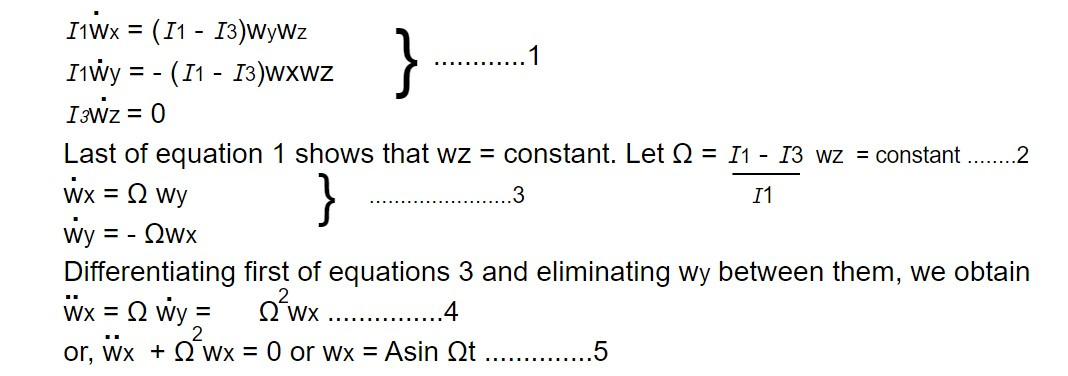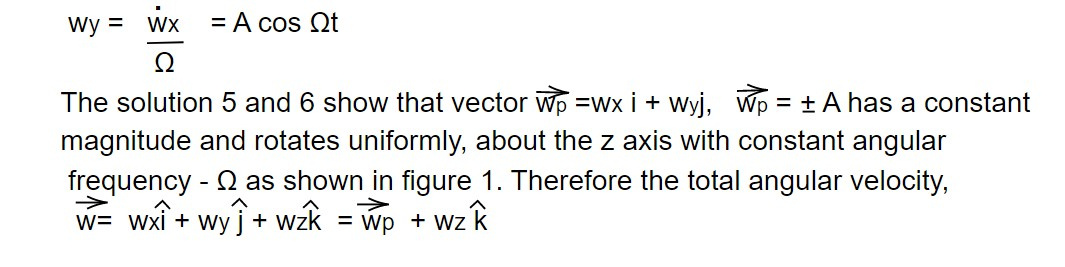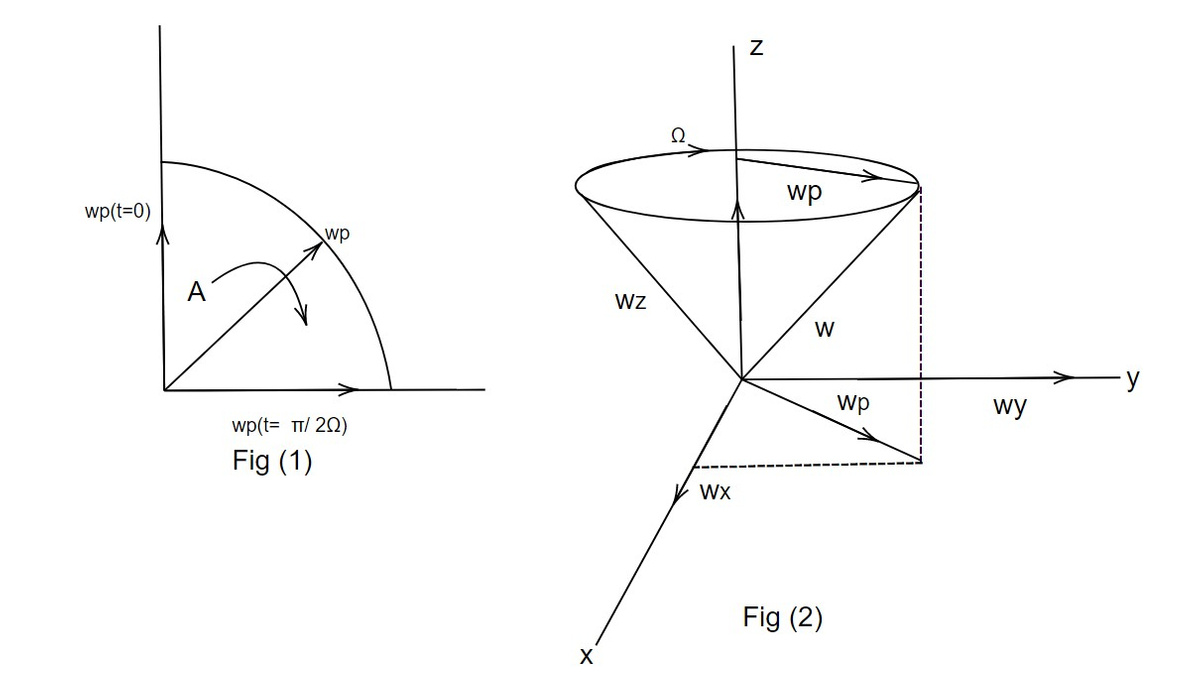# Force free motion of a symmetrical rigid body

Let us choose the symmetry axis as the principle z axis, so that I1 = I2 and Euler's equations simplifies towhere A is some constant and we have chosen initial condition wx = 0 when t= 0. We can find wy by combining (5) with first of equation (3); the result isis also constant in magnitude and precesses about the z axis with the same constant angular frequency Ω. Figure 2 shows how this precessional motion works, showing how w sweeps out a cone around the z axis with a constant angular frequency. From 2, it is clear that the precessional velocity will be slower than wz the closer I1 and I3 are to one another.

The results may be applied to rotating earth, for it may be considered to be torque free body. The earth is nearly symmetric about the polar axis and slightly bulged at the equator so that I3> I1. It is found that

I3 - I1 / I1 = 1/300 and wz = 2π/day

Hence the period of precession of the axis of rotation about the symmetry axis will be

T = 2π /Ω = 2π. I1 /wz (I3 - I1) = 300 days ≈ 10 monthsThis note is taken from Classical Mechanics, MSC physics, Nepal